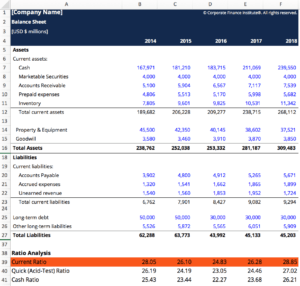# Current Ratio Calculator

## Current Ratio Calculator

This Current Ratio Calculator will help you calculate the current ratio given the sum of all current assets and current liabilities. The Current Ratio is a key liquidity ratio that measures the ability of the company to cover its short-term liabilities.

Here is a snippet of the current ratio calculator template.### Current Ratio formula

The Current Ratio formulas is:

Current Ratio = Current Assets / Current Liabilities

### Why use the Current Ratio?

The current ratio assesses the operations of a company and how financially solid the company is in relation to its outstanding debt. Knowing this ratio can be vital for decision-making of creditors, investors, and suppliers of a company. When it comes to these stakeholders, the current ratio is an important tool to aid them in their business decision.

### More Free Templates

For more resources, check out our business templates library to download numerous free Excel modeling, PowerPoint presentation, and Word document templates.

• Excel Modeling Templates
• PowerPoint Presentation Templates
• Transaction Document Templates
• Free eBooks

### Financial Analyst Certification

Become a certified Financial Modeling and Valuation Analyst (FMVA)® by completing CFI’s online financial modeling classes and training program!